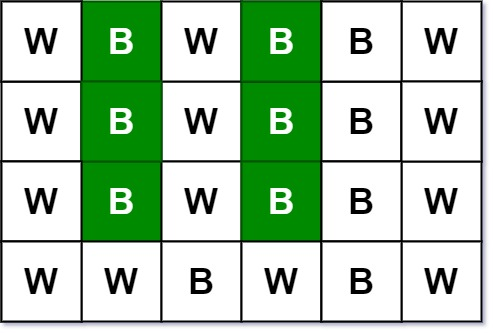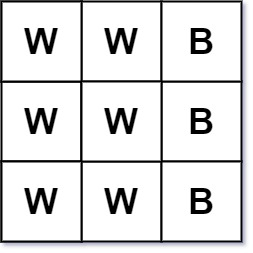### LeetCode• ㊗️
• 大家
• offer
• 多多！

## Problem

Given an m x n picture consisting of black ‘B’ and white ‘W’ pixels and an integer target, return the number of black lonely pixels.

A black lonely pixel is a character ‘B’ that located at a specific position (r, c) where:

• Row r and column c both contain exactly target black pixels.
• For all rows that have a black pixel at column c, they should be exactly the same as row r.

Example 1:Input: picture = [["W","B","W","B","B","W"],["W","B","W","B","B","W"],["W","B","W","B","B","W"],["W","W","B","W","B","W"]], target = 3
Output: 6
Explanation: All the green 'B' are the black pixels we need (all 'B's at column 1 and 3).
Take 'B' at row r = 0 and column c = 1 as an example:
- Rule 1, row r = 0 and column c = 1 both have exactly target = 3 black pixels.
- Rule 2, the rows have black pixel at column c = 1 are row 0, row 1 and row 2. They are exactly the same as row r = 0.


Example 2:Input: picture = [["W","W","B"],["W","W","B"],["W","W","B"]], target = 1
Output: 0


Constraints:

• m == picture.length
• n == picture[i].length
• 1 <= m, n <= 200
• picture[i][j] is ‘W’ or ‘B’.
• 1 <= target <= min(m, n)

## Code

[
["W","B","W","B","B","W"],
["B","W","B","W","W","B"],
["W","B","W","B","B","W"],
["B","W","B","W","W","B"],
["W","W","W","B","B","W"],
["B","W","B","W","W","B"]
]

target = 3

result is 9, not 13

class Solution {
public int findBlackPixel(char[][] picture, int target) {
int m = picture.length;
int n = picture.length;

Map<String, Integer> map = new HashMap<>();
// 每一列有多少B
int[] colCount = new int[n];

for (int i = 0; i < m; i++) {
int rowCount = 0;
StringBuilder sb = new StringBuilder();

for(int j = 0; j < n; j++) {
if (picture[i][j] == 'B') {
rowCount++;
colCount[j]++;
}

sb.append(picture[i][j]);
}

// 本行符合要求
if (rowCount == target) {
String str = sb.toString();
map.put(str, map.getOrDefault(str, 0) + 1);
}
}

int res = 0;

for (String rowStr : map.keySet()) {
// 所有有target个B的行 正好有target个
if (map.get(rowStr) == target) {
for (int j = 0; j < n; j++) {
if (rowStr.charAt(j) == 'B' && colCount[j] == target) {
res += target;
}
}
}
}

return res;
}
}